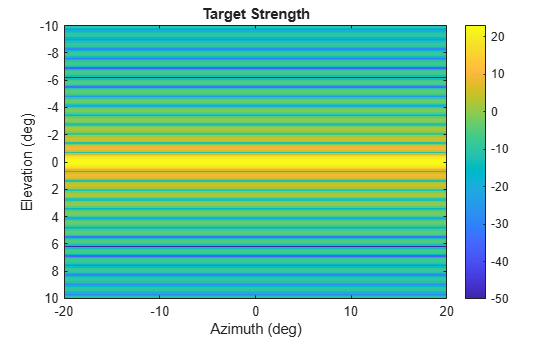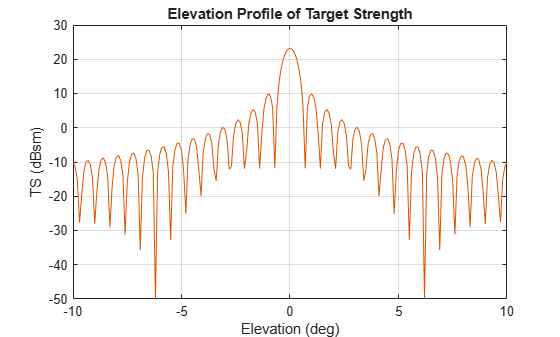# value

Target strength at specified angle and frequency

## Syntax

``tsval = value(tssig,az,el,freq)``

## Description

example

````tsval = value(tssig,az,el,freq)` returns the value, `tsval`, of the target strength specified by the target strength signature object, `tssig`, computed at azimuth `az`, elevation `el`, and frequency `freq`. If the specified azimuth and elevation is outside of the region in which the target strength signature is defined, the target strength value, `tsval`, is returned as `-Inf` in dBsm.```

## Input Arguments

expand all

Target strength signature, specified as a `tsSignature` object.

Azimuth angle, specified as scalar or length-M real-valued vector. Units are in degrees. The `az`, `el`, and `freq` arguments must have the same size. You can, however, specify one or two arguments as scalars, in which case the arguments are expanded to length-M.

Data Types: `double`

Elevation angle, specified as scalar or length-M real-valued vector. The `az`, `el`, and `freq` arguments must have the same size. You can, however, specify one or two arguments as scalars, in which case the arguments are expanded to length-M. Units are in degrees.

Data Types: `double`

TS frequency, specified as a positive scalar or length-M vector with positive, real elements. The `az`, `el`, and `freq` arguments must have the same size. You can, however, specify one or two arguments as scalars, in which case the arguments are expanded to length-M. Units are in Hertz.

Example: `20e3`

Data Types: `double`

## Output Arguments

expand all

Target strength, returned as a scalar or real-valued length-M vector. Units are in dBsm.

## Object Functions

 `perturbations` Perturbation defined on object `perturb` Apply perturbations to object

## Examples

expand all

Specify the target strength (TS) of a 5m long rigid cylinder immersed in water and plot TS values along an azimuth cut. Assume the short-wavelength approximation. The cylinder radius is 2m. The speed of sound is 1520 m/s.

```L = 5; a = 2;```

Create an array of target strengths at two wavelengths. First, specify the range of azimuth and elevation angles over which TS is defined. Then, use an analytical model to compute the target strength. Create an image of the TS.

```lambda = [0.12, .1]; c = 1520.0; az = [-20:0.1:20]; el = [-10:0.1:10]; ts1 = ts_cylinder(L,a,az,el,lambda(1)); ts2 = ts_cylinder(L,a,az,el,lambda(2)); tsdb1 = 10*log10(ts1); tsdb2 = 10*log10(ts2); imagesc(az,el,tsdb1) title('Target Strength') xlabel('Azimuth (deg)') ylabel('Elevation (deg)') colorbar```Create a `tsSignature` object and plot an elevation cut at $3{0}^{\circ }$ azimuth.

```tsdb(:,:,1) = tsdb1; tsdb(:,:,2) = tsdb2; freq = c./lambda; tssig = tsSignature('Pattern',tsdb,'Azimuth',az,'Elevation',el,'Frequency',freq); ts = value(tssig,30,el,freq(1)); plot(el,tsdb1) grid title('Elevation Profile of Target Strength') xlabel('Elevation (deg)') ylabel('TS (dBsm)')``````function ts = ts_cylinder(L,a,az,el,lambda) k = 2*pi/lambda; beta = k*L*sind(el')*ones(size(az)); gamma = cosd(el')*ones(size(az)); ts = a*L^2*(sinc(beta).^2).*gamma.^2/2/lambda; ts = max(ts,10^(-5)); end function s = sinc(theta) s = ones(size(theta)); idx = (abs(theta) <= 1e-2); s(idx) = 1 - 1/6*(theta(idx)).^2; s(~idx) = sin(theta(~idx))./theta(~idx); end```

## Version History

Introduced in R2018b